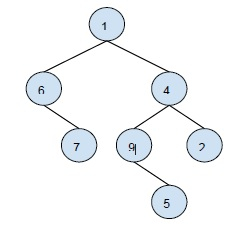# Print all the levels with odd and even number of nodes in it in C++

In this problem, we are given a tree. And we have to print all the levels with even number of nodes and odd number of nodes in it.

Let’s take an example to understand the concept betterOutput −

Levels with odd number of nodes: 1, 3, 4
Levels with even number of nodes: 2

Explanation − The first level has only 1 element(odd), 2nd level contains two elements(even), 3rd level contains 3 elements(odd) and 4th level contains 1 element(even).

Now, to solve this problem. We need to find the count of nodes at each level and print the even-odd levels accordingly.

We will follow the following steps to find the solution −

Step 1 − Run search algorithm for every level using height[node]=1+height[parent]

Step 2 − For every level store the number of nodes at that level.

Step 3 − iterate over the array containing elements, and print at even and odd levels.

## Example

Live Demo

#include <bits/stdc++.h>
using namespace std;
void traversal(int node, int parent, int height[], int vis[], vector<int> tree[]){
height[node] = 1 + height[parent];
vis[node] = 1;
for (auto it : tree[node]) {
if (!vis[it]) {
traversal(it, node, height, vis, tree);
}
}
}
void insert(int x, int y, vector<int> tree[]){
tree[x].push_back(y);
tree[y].push_back(x);
}
void evenOddLevels(int N, int vis[], int height[]){
int mark[N + 1];
memset(mark, 0, sizeof mark);
int maxLevel = 0;
for (int i = 1; i <= N; i++) {
if (vis[i])
mark[height[i]]++;
maxLevel = max(height[i], maxLevel);
}
cout << "The levels with odd number of nodes are: ";
for (int i = 1; i <= maxLevel; i++) {
if (mark[i] % 2)
cout << i << " ";
}
cout << "\nThe levels with even number of nodes are: ";
for (int i = 1; i <= maxLevel; i++) {
if (mark[i] % 2 == 0)
cout << i << " ";
}
}
int main(){
const int N = 9;
vector<int> tree[N + 1];
insert(1, 2, tree);
insert(1, 3, tree);
insert(2, 4, tree);
insert(2, 5, tree);
insert(5, 7, tree);
insert(5, 8, tree);
insert(3, 6, tree);
insert(6, 9, tree);
int height[N + 1];
int vis[N + 1] = { 0 };
height = 0;
traversal(1, 0, height, vis, tree);
evenOddLevels(N, vis, height);
return 0;
}

## Output

The levels with odd number of nodes are: 1 3 4
The levels with even number of nodes are: 2

Updated on: 17-Jan-2020

97 Views